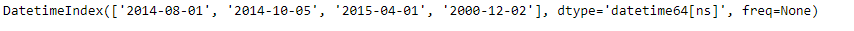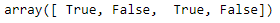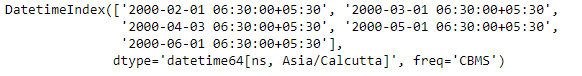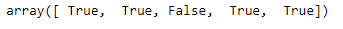Python | Pandas DatetimeIndex.is_month_start

• Last Updated : 24 Dec, 2018

Python is a great language for doing data analysis, primarily because of the fantastic ecosystem of data-centric python packages. Pandas is one of those packages and makes importing and analyzing data much easier.

Pandas DatetimeIndex.is_month_start attribute returns a numpy array containing logical values corresponding to each entries in the DatetimeIndex object. The value is set to True if the date is the first day of the month. Else the function returns False indicating the date is not the first day of the month.

Syntax: DatetimeIndex.is_month_start

Return: numpy array containing logical values.

Example #1: Use DatetimeIndex.is_month_start attribute to check if the dates present in the DatetimeIndex object is the first day of the month.

 # importing pandas as pdimport pandas as pd  # Create the DatetimeIndexdidx = pd.DatetimeIndex(['2014-08-01', '2014-10-05',                         '2015-04-01', '2000-12-02'])  # Print the DatetimeIndexprint(didx)

Output :Now we want to find if the dates contained in the given DatetimeIndex object is the first day of the month.

 # find if the days are the first day of the month.didx.is_month_start

Output :As we can see in the output, the function has returned a numpy array containing logical values for each entry of the DatetimeIndex object. True values indicate the corresponding date was the first day of the month and False value indicate the corresponding date was not the first day of the month.

Example #2: Use DatetimeIndex.is_month_start attribute to check if the dates present in the DatetimeIndex object is the first day of the month.

 # importing pandas as pdimport pandas as pd  # Create the DatetimeIndexdidx = pd.DatetimeIndex(start ='2000-01-10 06:30', freq ='CBMS',                               periods = 5, tz ='Asia/Calcutta')  # Print the DatetimeIndexprint(didx)

Output :Now we want to find if the dates contained in the given DatetimeIndex object is the first day of the month.

 # find if the days are the first day of the month.didx.is_month_start

Output :As we can see in the output, the function has returned a numpy array containing logical values for each entry of the DatetimeIndex object. True values indicate the corresponding date was the first day of the month and False value indicate the corresponding date was not the first day of the month.

Attention geek! Strengthen your foundations with the Python Programming Foundation Course and learn the basics.

To begin with, your interview preparations Enhance your Data Structures concepts with the Python DS Course. And to begin with your Machine Learning Journey, join the Machine Learning - Basic Level Course

My Personal Notes arrow_drop_up